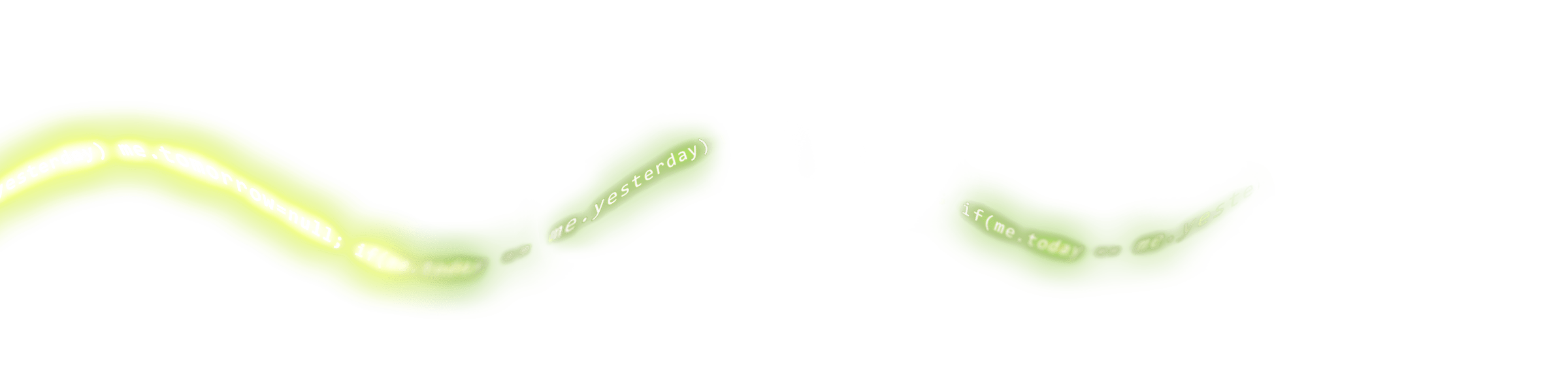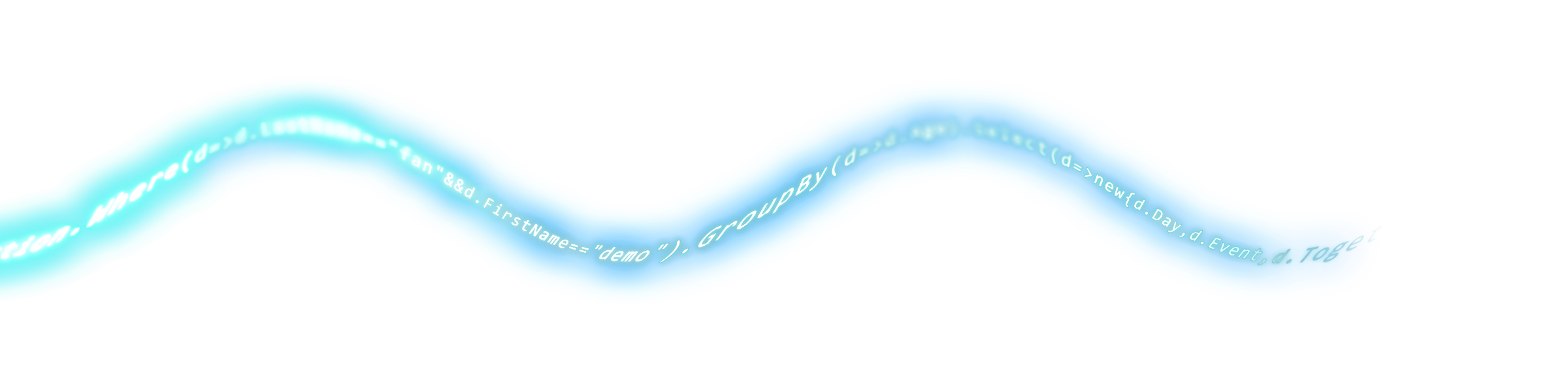# demoshop

••••••••demo, trying to be the best_

## 在LINQ中使用LIKE

• 2009-04-17
• 29153
• 0廢話不多說，直接來比較吧當我們利用以下語法來查詢

```(from p in db.物件資訊 where p.User2.Contains("北") select p)
```

```WHERE [t0].[User2] LIKE @p0',N'@p0 nvarchar(3)',@p0=N'%北%'
```

```(from p in db.物件資訊 where p.User2.Contains("北%") select p);
```

```WHERE [t0].[User2] LIKE @p0 ESCAPE ''~''',N'@p0 nvarchar(5)',@p0=N'%北~%%'
```

```(from p in db.物件資訊 where SqlMethods.Like(p.User2,"%北") select p);
```

`WHERE [t0].[User2] LIKE @p0',N'@p0 nvarchar(2)',@p0=N'%北'`只有Linq To Sql 才可以這樣用

```public static IQueryable<T> Like<T>(this IQueryable<T> source, string propertyName, string keyword)
{
var type = typeof(T);
var property = type.GetProperty(propertyName);
var parameter = Expression.Parameter(type, "p");
var propertyAccess = Expression.MakeMemberAccess(parameter, property);
var constant = Expression.Constant("%" + keyword + "%");
MethodCallExpression methodExp = Expression.Call(null, typeof(SqlMethods).GetMethod("Like", new Type[] { typeof(string), typeof(string) }), propertyAccess, constant);
Expression<Func<T, bool>> lambda = Expression.Lambda<Func<T, bool>>(methodExp, parameter);
return source.Where(lambda);
}
```http://msdn.microsoft.com/zh-tw/library/system.data.linq.sqlclient.sqlmethods.aspx

### 徽章區